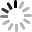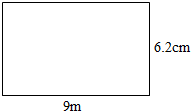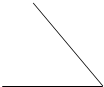4．学校组织看电影，小芳坐在二排4号，小欣坐在二排2号，小明与她俩坐在同一条直线上，并且坐在她俩的中间，小明坐在（　　）
 A． 四排2号 B． 二排3号 C． 五排2号年级 高中课程 年级 初中课程 高一 高一免费课程推荐！ 初一 初一免费课程推荐！ 高二 高二免费课程推荐！ 初二 初二免费课程推荐！ 高三 高三免费课程推荐！ 初三 初三免费课程推荐！ 更多初中、高中辅导课程推荐,点击进入>>

14．0.48读作零点四八，它里面48个0.01.6里面有60个0.1；不改变大小，把6改写成两位小数是6.00．

6．
 2000克=2千克 3t=3000kg 6吨=6000千克 3分=180秒 120分=2时 5时=300分

7．笑笑和淘气进行写数比赛．在○中填上合适的一位小数0＜○＜1，二人轮流写，每人每次写一个，不许和自己或他人写过的数重复，谁写的数多谁获胜．你认为这个比赛规则公平吗？为什么？

12．直接写得数．
 1.21×$\frac{3}{22}$= $\frac{1}{6}$×12= $\frac{2}{9}$÷$\frac{3}{5}$= $\frac{1}{2}$+$\frac{7}{12}$= （3$\frac{1}{8}$+$\frac{7}{9}$）×0= $\frac{2}{3}$-$\frac{2}{3}$×$\frac{4}{13}$= 1÷$\frac{1}{3}$= 1-$\frac{1}{2}$-$\frac{1}{3}$= $\frac{5}{8}$×$\frac{2}{3}$= $\frac{5}{6}$×（18+$\frac{6}{25}$）=

19．怎样算便怎样算：
$\frac{2}{5}$÷（$\frac{3}{4}$+$\frac{2}{5}$）       $\frac{7}{9}$÷$\frac{11}{5}$+$\frac{2}{9}$×$\frac{5}{11}$　　      （$\frac{1}{8}$+$\frac{1}{4}$）×4
$\frac{7}{10}$×$\frac{3}{7}$×$\frac{5}{6}$         $\frac{2}{3}$+（$\frac{4}{7}$+$\frac{1}{2}$）×$\frac{7}{25}$        2-$\frac{6}{13}$÷$\frac{9}{26}$-$\frac{2}{3}$．

9．分别求出长方形的周长和面积．16．量出下面的角．13．计算如图的体积和表面积．
（1）把一根长2米的长方体木料锯成1米长的两段，表面积增加了2平方分米，求这根木料原来的体积．
（2）一个长方体和一个正方体的棱长之和相等，已知长方体长、宽、高分别是6分米、4分米、2分米，求正方体体积．
（3）钢材长15分米，横截面面积是1.2平方分米．如果把它煅烧成一横截面面积是0.1平方分米的钢筋，求这根钢筋的长．